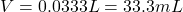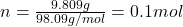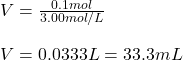What is the volume of 3.00 M sulfuric aid that contain 9.809 g of H2SO4 (98.09g/mol)

Question

What is the volume of 3.00 M sulfuric aid that contain 9.809 g of H2SO4 (98.09g/mol)

in progress 0
5 months 2021-08-18T06:42:54+00:00 1 Answers 4 views 0Explanation:

Hello there!

In this case, according to the given information, it is necessary for us remember that the molarity is calculated by dividing the moles by the volume of the solution in liters so the volume is calculated by dividing moles by the given molarity. However, we first need to calculate the mole given the mass and molar mass of the solute, H2SO4:Thus, the volume turns out:Regards!